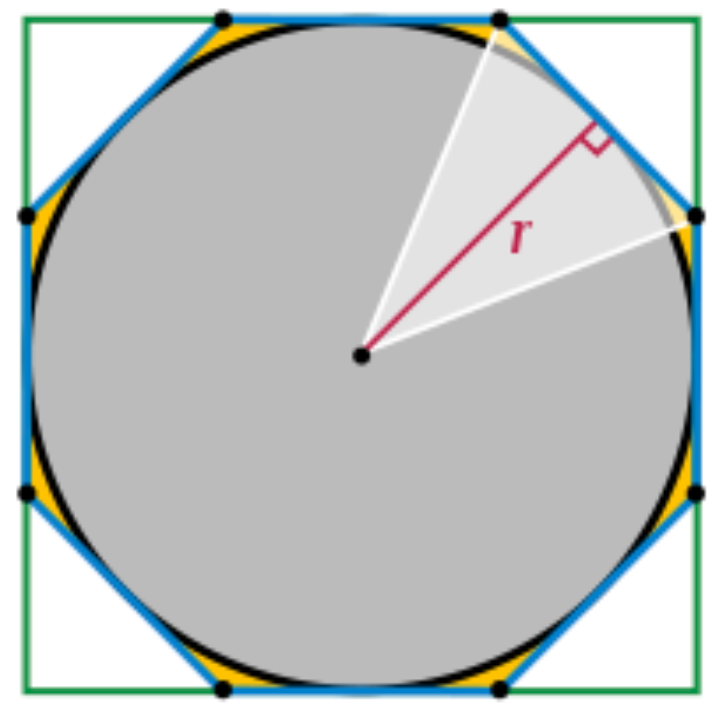top of page
Search

# A little History of pi.

3.14159265359. That’s pi. Well, some of it at least; an infinitesimal section of it, in fact.

Pi is an irrational non-terminating decimal, and a sort of magic number in mathematics: it is the ratio of a circle’s circumference to its diameter. This seems trivial enough, but what’s really special about it, is that it is universal for every circle of any dimension. Its uncanny reach extends to countless natural phenomenons; the spirals of a DNA double helix, meanders in a river, the shape of water ripples— pi is everywhere— and that is why every year, on March the 14th, (3/14 in an American calendar) we honor this elusive constant, which has shaped the world’s geometry since before we even knew it.

How did we discover pi? Well, as with most mathematical breakthroughs, the Babylonians and the Egyptians seemed to have a good idea of what it was about— they calculated it to be 3.125 and 3.16 respectively—but it wasn’t until Archimedes, deemed one of the greatest minds of all time, that an accurate estimate was found.

Archimedes determined that pi lay between 3.1408 and 3.1429 using a method of approximation by infinite series, a method which was replicated by Runnymede students on our pi day celebration this year. The way he originally did this was by first noting that a regular polygon drawn on the inside and on the outside of a circle would have a perimeter similar to the circumference of the circle. The two values would create an inequality in which the value for the circumference would lie.

He knew of the relationship between the diameter and the circumference dictated by a constant to be found, so he stared by inscribing a hexagon in a circle of diameter one, calculating the perimeter to be 3. He then drew another hexagon on the outside of the circle, and found its perimeter to be of about 3.46— which meant that the circumference of the circle had to be between 3 and 3.46— and hence the value of pi (because of using a 1: x ratio) also had to be between these values. He replicated this same method using polygons with a larger number of sides, 12, 24, 48, until finally reaching a whopping 96 sides, with which he obtained the inequality shown above; which is still true today. In fact, many computer based calculations of pi use the same method as Archimedes did, but with the gift of modern algorithm technology at their sides.It is often said that precision is the key to scientific progress, and the next mathematician to make strides in finding a more precise value for pi was Zhu Chongzhi, who through the same method of approximation by infinite series, created a 12,288-sided polygon, and found pi correct to five decimal places in about 450 AD.

It was not until the 15th Century in which the challenge of finding pi was taken up by yet another brilliant mathematician, Madhava of Sangamagrama, an Indian astronomer and intellectual considered to be the founder of the Kerala school. While Europe was still suffering the end of the Dark Ages, Asia was a cradle for pioneering and innovation; and Madhava was stark proof of this. Other than finding pi to 11 decimal places (through the binomial expansion of an infinite series known as the Madhava-Leibniz series) he also dabbled in creating series approximations to trigonometric functions. His greatest contribution was perhaps to the concept of infinity however, as he was one of the first to acknowledge the possibility of non-terminating irrational numbers, which is, in essence, what pi is.

In the 18th century pi was linked to yet another feature of mathematics— probability— by mathematician Georges-Louis Leclerc, the Count of Buffon. Buffon’s needle problem, which our students also carried out themselves this pi day, consists of the following: say you have a flat surface with equally spaced out parallel lines drawn on it, with separation t between them, given a needle of length l what is the probability the needle will land crossing a line?

It seems simple enough, if you drop the needle enough times, you can record how many times it crosses a line and go from there; but this problem results in very complicated calculus mixed in with probability density functions (and yes, it is as nasty as it sounds). Italian mathematician Lazzarini managed to simplify this problem with a formula which obtained a straightforward approximation to pi. Our students discovered themselves that two times the length of the needle, multiplied by the number of needle tosses, all divided by the separation between parallels and the number of times the needles crossed a line would give them a mostly accurate approximation to pi, demonstrating how transcendental this constant really is.To this day, the most accurate approximation of pi was, expectedly, made by a computer. It was this year on pi day, on which Google partnered with Emma Haruka Iwao, a Japanese mathematician and computer scientist, to find pi accurate to 31,415,926,535,897 digits, a number so astounding I don’t even know how to put into words. Her predecessors would have marvelled at the vastness of this achievement.

And as we often say in maths; now, I know pi.

Beatriz J, Year 12